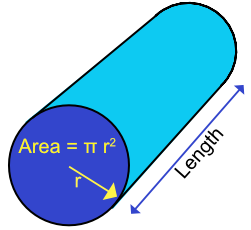Volume of a Cylinder

## Volume of a Cylinder

A cylinder is a circular prism. As with other prisms, the volume of a cylinder can be calculated using the cross-sectional area x length.

Th cross-section of a cylinder is a circle. As the area of a circle =πr^2, the volume of a cylinder is πr^2l, where r is the radius of the circular end, and l is the length of the cylinder.## Example 1

What is the volume of a cylinder with a diameter of 4cm and a length of 42cm? Give your answer correct to 1 decimal place.

 For a circle: Area = pir^2 Substitute A = pi xx 2^2 = 4pi

The cross-sectional area is 4pi

 For a cylinder: Volume = area xx length Substitute V = 4pi xx 42 = 527.79

 For a Cylinder Volume = pir^2l Substitute 1000 = pir^2 xx 100 Divide both sides by 100 10 = pir^2 Divide both sides by pi 3.183 = r^2 Square root 1.784 = r